#### Free Electron Theory of Metals, Physics tutorial

Introduction:

The free electron theory of metals points out to the case in which the atomic valance electrons are treated as if they are free instead of being bound to the lattice points. Our supposition amounts to assuming that the electrons move in a uniform potential instead of the true periodic potential given by the positive ions. The fundamental supposition of the theory is that a metal is equivalent to the gas of free electrons in an otherwise empty box.

Definition:

The free electron model is the easiest means to represent the electronic structure of the metals.

Free electron model:

A free electron model is the easiest means to symbolize the electronic structure of metals. However the free electron model is a great oversimplification of the reality, surprisingly in most of the cases it works fairly well, so that it is capable to explain numerous significant properties of metals. According to this model, the valence electrons of the constituent atoms of the crystal become conduction electrons and travel freely all through the crystal. Thus, within this model we avoid the interaction of conduction electrons by ions of the lattice and the interaction between the conduction electrons. In this sense we are talking regarding a free electron gas. Though, there is a principle difference between the free electron gas and ordinary gas of molecules. At first, electrons are charged particles. Thus, in order to maintain the charge neutrality of the entire crystal, we require comprising positive ions. This is done in the jelly model, according to which the positive charge of ions is smeared out equally all through the crystal. This positive background maintains the charge neutrality however doesn't apply any field on the electrons. Ions make a uniform jelly to which electrons move. Second significant property of the free electron gas is that it must meet up the Pauli Exclusion Principle that leads to significant effects.

One-dimension:

We at first take a free electron gas in one dimension. We suppose that an electron of mass 'm' is confined to a length 'L' by infinite potential barriers. The wave function Ψn(x) of the electron is a solution of the Schrodinger equation, HΨ(x) = EΨ(x), here En is the energy of electron in the orbital. As 'w' can  be suppose that the potential lies at zero, the Hamiltonian 'H' comprises only the kinetic energy in such a way that,

n(x) = (p2/2m)Ψn(x) = EnΨn(x)

It will be noted that this is a one-electron equation, which signifies that we neglect the electron-electron interactions. We utilize the word orbital to illustrate the solution of this equation.

As Ψn(x)is a continuous function and is equivalent to zero beyond the length 'L', the boundary conditions for the wave function are Ψn(0) = Ψn(L) = 0. The solution of the above equation is thus:

Ψn(x) = A sin (πn/L)

Here 'A' is a constant and 'n' is an integer.

Such solutions correspond to standing waves having a different number of nodes in the potential well as is shown in the figure given below: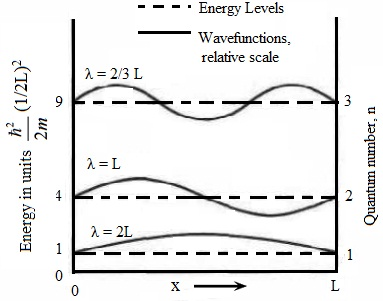Now we necessitate accommodating 'N' valence electrons in such quantum states. According to the Pauli Exclusion Principle no two electrons can contain their quantum number similar. That is, each and every electronic quantum state can be occupied via at most one electron. The electronic state in a 1-D solid is characterized through two quantum numbers that are n and ms, here 'n' is the positive integer and ms is the magnetic quantum number such that  ms = ± 1/2 according to the spin orientation.

Thus, each orbital labeled via the quantum number 'n' can accommodate two electrons, one by spin up and one by spin down orientation.

Let nF represent the highest filled energy level, where we begin filling the levels from the bottom (n = 1) and carry on filling higher levels by electrons till all 'N' electrons are accommodated. This is suitable to assume that 'N' is an even number. The condition 2nF = N finds out nF, the value of 'n' for the uppermost filled level.

The energy of the highest occupied level is termed as the Fermi energy EF. For one dimensional system of 'N' electrons we determine, by using the above equation:

EF = [(h/2π)2/2m] (πN/2L)2

In metals, the value of Fermi energy is of the order of 5 eV.

The ground state of the 'N' electron system is described in the first part of the figure shown below. All the electronic levels are filled up to the Fermi energy. All the levels above are unfilled.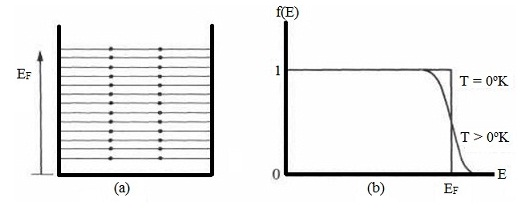The Fermi distribution:

It is the ground state of 'N' electron system at absolute zero. What happens if the temperature is raised? The kinetic energy of the electron gas rises with temperature. Thus, some energy levels become occupied that were empty at zero temperature, and some levels become vacant that were occupied at absolute zero. The distribution of electrons between the levels is generally explained by the distribution function, f(E), that is stated as the probability which the level E is occupied via an electron. Thus if the level is certainly empty, then, f(E) = 0, whereas if it is certainly full, then f(E) = 1. In common, f(E) consists of a value between zero and unity.

It follows from the previous discussion that the distribution functions for electrons at T = 0°K comprises the form:

f(E) = {1, E < EF

0, E < EF

That is, all the levels beneath EF are fully filled, and all those above EF are totally empty. This function is represented in the second part of the figure above that represents the discontinuity at the Fermi energy.

If the system is heated (T > 0°K), thermal energy excites the electrons. Though, all the electrons do not share this energy uniformly, as would be the case in the classical treatment, as the electrons lying well beneath the Fermi level EF can't absorb energy. If they did so, they would move to a higher level, which would be already occupied, and therefore the exclusion principle would be violated.

Remember in this context that the energy which an electron might absorb thermally is of the order kBT (= 0.025 eV at room temperature), that is much smaller than EF, this being of the order of 5 eV. Thus only those electrons close to the Fermi level can be excited, as the levels above EF are empty, and therefore whenever such electrons move to a higher level there is no violation of the exclusion principle. Therefore only such electrons - which are a small fraction of the total number - are capable of being thermally excited.

The distribution function at non-zero temperature is represented by the Fermi distribution function. The Fermi distribution function finds out the probability that an orbital of energy 'E' is occupied at thermal equilibrium:

f(E) = 1/{e(E-μ)/kBT + 1}

This function is as well illustrated in the second part of the above figure that shows that it is substantially similar as the distribution at T = 0°K, apart from very close to the Fermi level, where some of the electrons are excited from beneath EF to above it.

The quantity 'µ' is termed as the chemical potential. The chemical potential can be found out in a way that the total number of electrons in the system is equivalent to 'N'. At absolute zero µ = EF.

Three dimensions:

The Schrodinger equation in the (3-D) three dimensions takes the form: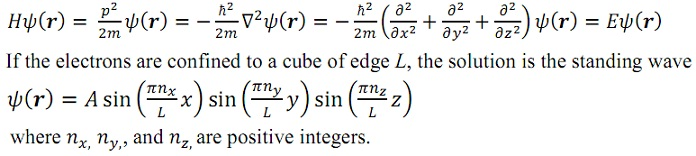In most of the cases, though, it is convenient to introduce periodic boundary conditions, as we did for phonons. The benefit of this explanation is that we suppose that our crystal is infinite and disregard the affect of the outer boundaries of the crystal on the solution. We need then that our wave function is periodic in x, y, and z directions having period L, in such a way that:

Ψ(x + L, y, z) = Ψ(x, y, z)

And likewise for the y and z coordinates. The solution of the Schrodinger equation that satisfies these boundary conditions consists of the form of the traveling plane wave:

Ψk(r) = Aexp(ik.r),

given that the component of the wave vector 'k' are determined from:

kx = 2πnx/L; ky = 2πny/L; kz = 2πnz/L;

Here nx, ny, and nz are the positive or negative integers.

If we now replace this solution to the Schrodinger equation we obtain for the energies of the orbital having the wave vector 'k'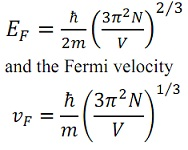The Fermi energy and the Fermi wave vector (that is, momentum) are found out by the number of valence electrons in the system. In order to determine the relationship between N and kF, we require to count the total number of orbital's in a sphere of radius kF that must be equivalent to N. There are two available spin states for a particular set of kx, ky, and kz. The volume in the k space that is occupies by this state is equivalent to: (2π/L)3. Therefore in the sphere of (4πkF3/3) the total number of states is:

2[(4πkF3/3)/(2π/L)3] = (V/3π2)kF3 = N

Here the factor 2 comes from the spin degeneracy. Then,

kF = (3π2N/V)1/3

This depends only of the particle concentration. We acquire then for the Fermi energy: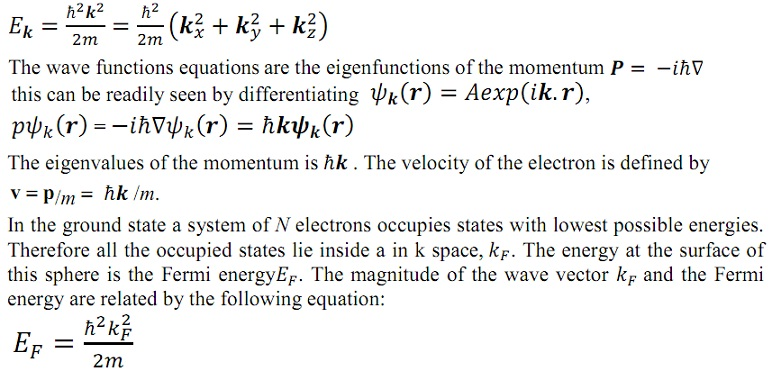The significant quantity that characterizes electronic properties of a solid is the density of states that is the number of electronic states per unit energy range. To determine it we utilize the equation of Fermi energy and write the total number of orbital's of energy < E:

N(E) = (V/3π2)[2me/(h/2π)2]3/2

The density of states is then:

D(E) = dN/dE = (V/2π2)[2m/(h/2π)2]3/2 .E1/2

Or equivalently,

D(E) = 3N/2E

Therefore within a factor of the order of unity, the number of states per unit energy interval at the Fermi energy, D(EF), is the total number of conduction electrons divided by the Fermi energy.

The density of states normalized in such a manner that the integral:

N = oEF D(E) dE

provides the total number of electrons in the system. At non-zero temperature we must take into account the Fermi distribution function in such a way that:

N = o D(E)f(E)dE

This expression as well finds out the chemical potential.

Heat capacity:

The question which caused the greatest difficulty in the early growth of the electron theory of metals concerns the heat capacity of the conduction electrons. Classical statistical mechanics forecasts that a free particle must encompass a heat capacity of 3/2kB, here kB is the Boltzmann constant. If 'N' atoms each provide one valence electron to the electron gas, and the electrons are freely mobile, then the electronic contribution to the heat capacity must be 3/2NkB, just as for the atoms of a monatomic gas. However the observed electronic contribution at room temperature is generally less than 0.01 of this value.

This discrepancy was resolved only on the discovery of the Pauli Exclusion Principle and the Fermi distribution function. Whenever we heat the specimen from absolute zero not every electron gains an energy ~ kBT as expected classically, however only such electrons, which encompass the energy within an energy range kBT of the Fermi level, can be excited thermally. Such electrons gain an energy that is itself of the order of kBT. This gives a qualitative solution to the problem of the heat capacity of the conduction electron gas. If 'N' is the total number of electrons, only a fraction of the order of kBT/EF can be excited thermally at temperature 'T', because only these lie within an energy range of the order of kBT of the top of the energy distribution.

Each of these NkBT/EF electrons consists of a thermal energy of the order of kBT. The total electronic thermal kinetic energy 'U' is of the order of U ≈ (NkBT/EF) kBT. The electronic heat capacity is Cel = dU/dT ≈ NkB(kBT/EF) and is directly proportional to T, in agreement by the experimental outcomes illustrated in the following section. At room temperature 'C' is smaller than the classical value ≈ NkB through a factor 0.01 or less.

We now derive a quantitative expression for the electronic heat capacity valid at low temperatures kBT << EF. The total energy of a system of 'N' electrons at temperature 'T' is:

U = o ED (E) f (E, T) dE,

Here f(E,T) is the Fermi distribution function and D(E) is the density of states. The heat capacity can be determined by differentiating the equation with respect to the temperature. Since only the distribution function depends on temperature we obtain:

Cel = o (E - Ef) D(E) [df(E, T)/dT] dE

We can as well represent the equation:

0 = EF (dN/dT) = EF o D(E) [df(E, T)/dT] dE

As we are interested only temperatures for which kBT << EF the derivative df/dT is large only at the energies that lie much close to the Fermi energy. Thus, we can ignore the variation of D(E) beneath the integral and take it outside the integrand at the Fermi energy, in such a way that,

Cel = D(EF) o(E - Ef) [df(E, T)/dT] dE

We as well ignore the variation of the chemical potential by the temperature and suppose that µ = EF that is good approximation at room temperature and below. Then,

df(E, T)/dT = [(E - EF)/kBT2]/[e[(E -EF)/kBT]]2

The equation Cel = D(EF) o(E - Ef) [df(E, T)/dT] dE can then be rewritten as:

Cel = D(EF) o [(E - EF)2/kBT2] [e(E - EF/kBT)/e(E - EF/kBT) +1]2 dE

Cel = D(EF) -EF/kBT [x2(kBT)3/kBT2] [ex/(ex + 1)2] dx

Taking into account that EF >> kBT, we can place the low integration limit to minus infinity and get:

Cel = D(EF)kB2T -∞ [x2ex/(ex + 1)2] dx = (π2/3)D(EF)kB2T

For a free electron gas we must utilize the equation D(E) = 3N/2E for the density of states to finally get:

Cel = (π2/2)NkB (T/TF),

Here we stated the Fermi temperature TF = EF/kB.

Experimentally the heat capacity at temperatures much beneath both the Debye temperature and the Fermi temperature can be symbolized in the form:

C = Cel + Cph = αT + βT3

The electronic word is dominant at adequately low temperatures. The constants α and β can be obtained via fitting the experimental data.

Tutorsglobe: A way to secure high grade in your curriculum (Online Tutoring)